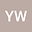•We study the large time behavior of solutions to the semilinear wave equation with space-dependent damping and absorbing nonlinearity in the whole space or exterior domains. Our result shows how the amplitude of the damping coefficient, the power of the nonlinearity, and the decay rate of the initial data at the spatial infinity determine the decay rates of the energy and the $L^2$-norm of the solution. In Appendix, we also give a survey of basic results on the local and global existence of solutions and the properties of weight functions used in the energy method.